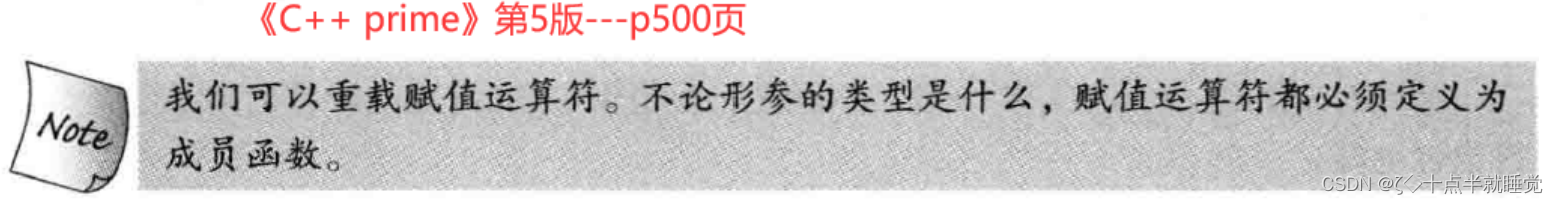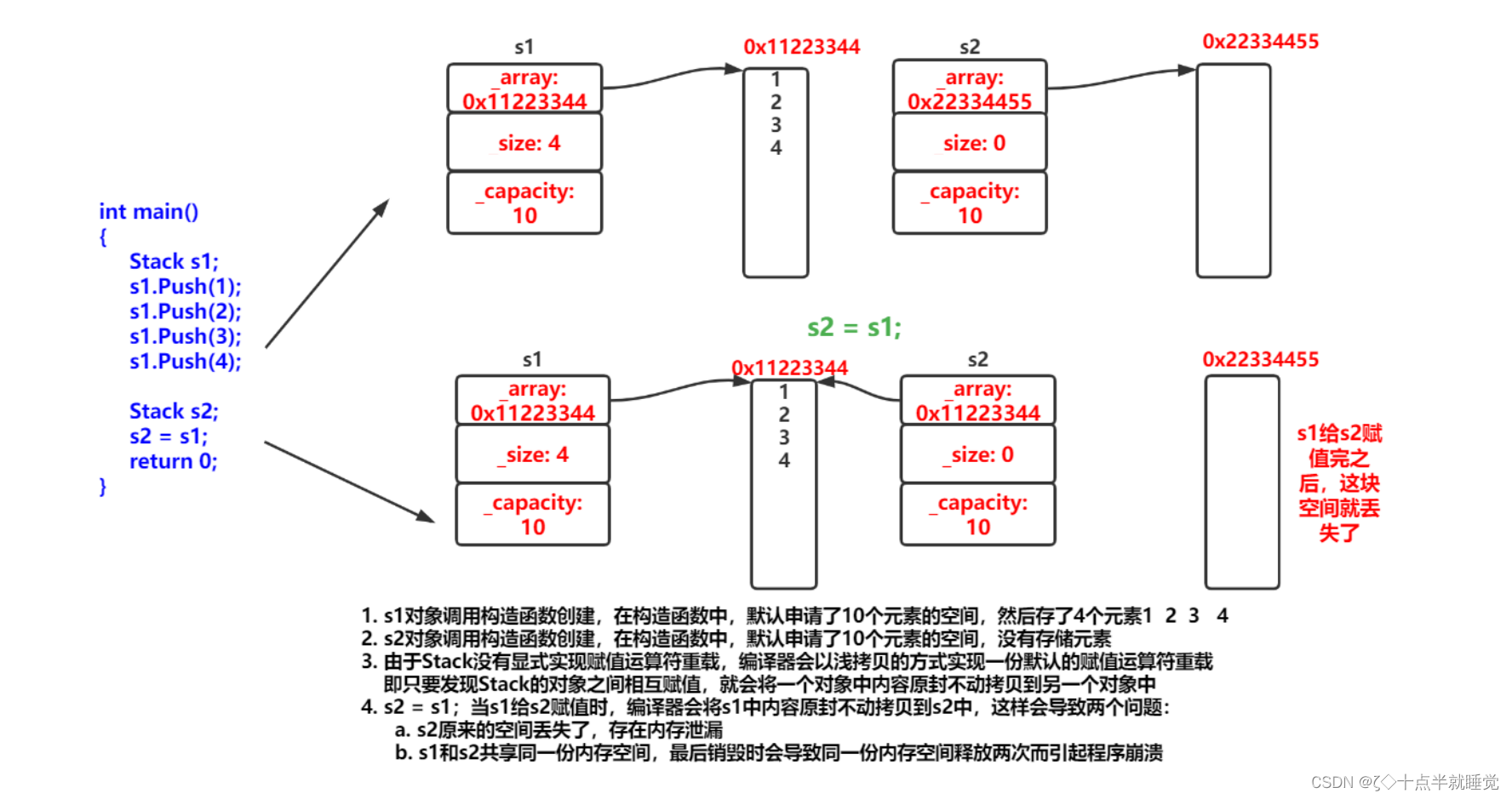# 【C++】运算符重载

## 运算符重载

C++为了增强代码的可读性引入了运算符重载，运算符重载是具有特殊函数名的函数，也具有其返回值类型，函数名以及参数列表。其返回值类型和参数列表与普通的函数类型。

nodejs

• 不能通过其他符号来创建新的操作符：比如`operator@`
• 重载操作符必须有一个类类型参数
• 用于内置类型的操作符其含义不能改变，例如：内置的整形+，不能改变其含义
• 作为类成员函数重载时，其形参看起来必操作数目少1，因为成员函数的第一个参数为隐藏的this
• `.* :: sizeof ?: .`注意以上5个运算符不能重载
``````// 全局的operator==
class Date
{
public:
Date(int year = 1900, int month = 1, int day = 1)
{
_year = year;
_month = month;
_day = day;
}
//private:
int _year;
int _month;
int _day;
};
// 这里会发现运算符重载成全局的就需要成员变量是公有的，那么问题来了，封装性如何保证？
// 这里其实可以用我们后面学习的友元解决，或者干脆重载成成员函数。
bool operator==(const Date& d1, const Date& d2)
{
return d1._year == d2._year
&& d1._month == d2._month
&& d1._day == d2._day;
}
void Test()
{
Date d1(2018, 9, 26);
Date d2(2018, 9, 27);
cout << (d1 == d2) << endl;
}
``````
``````class Date
{
public:
Date(int year = 1900, int month = 1, int day = 1)
{
_year = year;
_month = month;
_day = day;
}

// bool operator==(Date* this, const Date& d2)
// 这里需要注意的是，左操作数是this，指向调用函数的对象
bool operator==(const Date& d2)
{
return _year == d2._year
&& _month == d2._month
&& _day == d2._day;
}
private:
int _year;
int _month;
int _day;
};
``````

### 赋值运算符重载

1. 赋值运算符重载格式
• 参数类型`const T&`，传递引用可以提高传参效率
• 返回值类型`T&`，返回引用可以提高返回的效率，有返回值是为了支持连续赋值
• 检测是否自己给自己赋值
• 返回`*this`：要符合连续赋值的含义
``````class Date {
public:
Date(int year, int month, int day) {
_year = year;
_month = month;
_day = day;
}
Date(const Date& d) {
_year = d._year;
_month = d._month;
_day = d._day;
}
Date& operator=(const Date& d) {
if (this != &d) {
_year = d._year;
_month = d._month;
_day = d._day;
}
return *this;
}
private:
int _year;
int _month;
int _day;
};
``````
1. 赋值运算符只能重载成类的成员函数不能重载成全局函数
``````class Date
{
public:
Date(int year = 1900, int month = 1, int day = 1)
{
_year = year;
_month = month;
_day = day;
}
int _year;
int _month;
int _day;
};
// 赋值运算符重载成全局函数，注意重载成全局函数时没有this指针了，需要给两个参数
Date& operator=(Date& left, const Date& right)
{
if (&left != &right)
{
left._year = right._year;
left._month = right._month;
left._day = right._day;
}
return left;
}
// 编译失败：
// error C2801: “operator =”必须是非静态成员
``````3. 用户没有显式实现的时候，编译器会生成一个默认赋值运算符重载，以值的方式逐字节拷贝注意：内置成员类型变量是直接赋值的，而自定义类型成员变量需要调用对应类的赋值运算符重载完成赋值

``````class Time
{
public:
Time()
{
_hour = 1;
_minute = 1;
_second = 1;
}
Time& operator=(const Time& t)
{
if (this != &t)
{
_hour = t._hour;
_minute = t._minute;
_second = t._second;
}
return *this;
}
private:
int _hour;
int _minute;
int _second;
};
class Date
{
private:
// 基本类型(内置类型)
int _year = 1970;
int _month = 1;
int _day = 1;
// 自定义类型
Time _t;
};
int main()
{
Date d1;
Date d2;
d1 = d2;
return 0;
}
``````### 前置++和后置++重载

``````class Date
{
public:
Date(int year = 1900, int month = 1, int day = 1)
{
_year = year;
_month = month;
_day = day;
}
// 前置++：返回+1之后的结果
// 注意：this指向的对象函数结束后不会销毁，故以引用方式返回提高效率
Date& operator++()
{
_day += 1;
return *this;
}
// 后置++：
// 前置++和后置++都是一元运算符，为了让前置++与后置++形成能正确重载
// C++规定：后置++重载时多增加一个int类型的参数，但调用函数时该参数不用传递，编译器
//自动传递
// 注意：后置++是先使用后+1，因此需要返回+1之前的旧值，故需在实现时需要先将this保存
//一份，然后给this + 1
//       而temp是临时对象，因此只能以值的方式返回，不能返回引用
Date operator++(int)
{
Date temp(*this);
_day += 1;
return temp;
}
private:
int _year;
int _month;
int _day;
};
int main()
{
Date d;
Date d1(2022, 1, 13);
d = d1++;    // d: 2022,1,13   d1:2022,1,14
d = ++d1;    // d: 2022,1,15   d1:2022,1,15
return 0;
}
``````